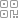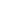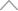6 0 7 3 8 1 1

22个案例详解Pandas数据分析/预处理时的实用技巧，超简单

• Pandas计算交叉列表
• Pandas将字符串与数值转化成时间类型
• Pandas将字符串转化成数值类型

Pandas当中的交叉列表

pandas.crosstab(index, columns, values=None, rownames=None, colnames=None, aggfunc=None, margins=False, margins_name='All', dropna=True, normalize=False)

• index: 指定了要分组的类目，作为行
• columns: 指定了要分组的类目，作为列
• rownames/colnames: 行/列的名称
• aggfunc: 指定聚合函数
• values: 最终在聚合函数之下，行与列一同计算出来的值
• normalize: 标准化统计各行各列的百分比

import pandas as pd df = pd.read_excel( io="supermarkt_sales.xlsx", engine="openpyxl", sheet_name="Sales", skiprows=3, usecols="B:R", nrows=1000, )

output

pd.crosstab(df['城市'], df['顾客类型'])

output

pd.crosstab(df['省份'], [df['顾客类型'], df["性别"]])

output

pd.crosstab(df['省份'], df['顾客类型'], colnames = ['顾客的类型'], rownames = ['各省份名称'])

output

pd.crosstab(df['省份'], df['顾客类型'], margins = True)

output

pd.crosstab(df['省份'], df['顾客类型'], margins = True, margins_name="汇总")

output

pd.crosstab(df['省份'], df['顾客类型'], normalize=True)

output

pd.crosstab(df['省份'], df['顾客类型'], normalize=True).style.format('{:.2%}')

output

pd.crosstab(df['省份'], df['顾客类型'], values = df["总收入"], aggfunc = "mean")

output

df_1 = pd.crosstab(df['省份'], df['顾客类型'], values=df["总收入"], aggfunc="mean").round(2)

output

时间类型数据的转化

df = pd.DataFrame({'date': [1470195805, 1480195805, 1490195805], 'value': [2, 3, 4]}) pd.to_datetime(df['date'], unit='s')

output

0 2016-08-03 03:43:25 1 2016-11-26 21:30:05 2 2017-03-22 15:16:45 Name: date, dtype: datetime64[ns]

df = pd.DataFrame({'date': [1470, 1480, 1490], 'value': [2, 3, 4]}) pd.to_datetime(df['date'], unit='D')

output

0 1974-01-10 1 1974-01-20 2 1974-01-30 Name: date, dtype: datetime64[ns]

pd.to_datetime('2022/01/20', format='%Y/%m/%d')

output

Timestamp('2022-01-20 00:00:00')

pd.to_datetime('2022/01/12 11:20:10', format='%Y/%m/%d %H:%M:%S')

output

Timestamp('2022-01-12 11:20:10')

• %y 两位数的年份表示(00-99)
• %Y 四位数的年份表示(000-9999)
• %m 表示的是月份(01-12)
• %d 表示的是一个月当中的一天(0-31)
• %H 表示的是24小时制的小时数
• %I 表示的是12小时制的小时数
• %M 表示的是分钟数 (00-59)
• %S 表示的是秒数(00-59)
• %w 表示的是星期数，一周当中的第几天，从星期天开始算
• %W 表示的是一年中的星期数

df = pd.DataFrame({'date': ['3/10/2000', 'a/11/2000', '3/12/2000'], 'value': [2, 3, 4]}) # 会报解析错误 df['date'] = pd.to_datetime(df['date'])

output

df['date'] = pd.to_datetime(df['date'], errors='ignore') df

output

date value 0 3/10/2000 2 1 a/11/2000 3 2 3/12/2000 4

df['date'] = pd.to_datetime(df['date'], errors='coerce') df

output

date value 0 2000-03-10 2 1 NaT 3 2 2000-03-12 4

数值类型的转换

df = pd.DataFrame({ 'string_col': ['1','2','3','4'], 'int_col': [1,2,3,4], 'float_col': [1.1,1.2,1.3,4.7], 'mix_col': ['a', 2, 3, 4], 'missing_col': [1.0, 2, 3, np.nan], 'money_col': ['£1,000.00','£2,400.00','£2,400.00','£2,400.00'], 'boolean_col': [True, False, True, True], 'custom': ['Y', 'Y', 'N', 'N'] })

output

df.dtypes

output

string_col object int_col int64 float_col float64 mix_col object missing_col float64 money_col object boolean_col bool custom object dtype: object

df.info()

output

<class 'pandas.core.frame.DataFrame'> RangeIndex: 4 entries, 0 to 3 Data columns (total 8 columns): # Column Non-Null Count Dtype --- ------ -------------- ----- 0 string_col 4 non-null object 1 int_col 4 non-null int64 2 float_col 4 non-null float64 3 mix_col 4 non-null object 4 missing_col 3 non-null float64 5 money_col 4 non-null object 6 boolean_col 4 non-null bool 7 custom 4 non-null object dtypes: bool(1), float64(2), int64(1), object(4) memory usage: 356.0+ bytes

df['string_col'] = df['string_col'].astype('int') df.dtypes

output

string_col int32 int_col int64 float_col float64 mix_col object missing_col float64 money_col object boolean_col bool custom object dtype: object

df['string_col'] = df['string_col'].astype('float') df.dtypes

output

string_col float64 int_col int64 float_col float64 mix_col object missing_col float64 money_col object boolean_col bool custom object dtype: object

df['mix_col'] = df['mix_col'].astype('int')

output

output

df.dtypes

output

string_col float64 int_col int64 float_col float64 mix_col float64 missing_col float64 money_col object boolean_col bool custom object dtype: object

"mix_col"这一列的数据类型被转换成了float64类型，要是我们想指定转换成我们想要的类型，例如

df['mix_col'] = pd.to_numeric(df['mix_col'], errors='coerce').astype('Int64') df['mix_col'].dtypes

output

Int64Dtype()

df['money_replace'] = df['money_col'].str.replace('£', '').str.replace(',','') df['money_replace'] = pd.to_numeric(df['money_replace']) df['money_replace']

output

0 1000.0 1 2400.0 2 2400.0 3 2400.0 Name: money_replace, dtype: float64

df['money_regex'] = df['money_col'].str.replace('[£,]', '', regex=True) df['money_regex'] = pd.to_numeric(df['money_regex']) df['money_regex']

df = df.astype({ 'string_col': 'float16', 'int_col': 'float16' })

df = pd.read_csv( 'dataset.csv', dtype={ 'string_col': 'float16', 'int_col': 'float16' } )

新知精选

优选课程 新知学院

•扫码下载商业新知APP~

•回到顶部